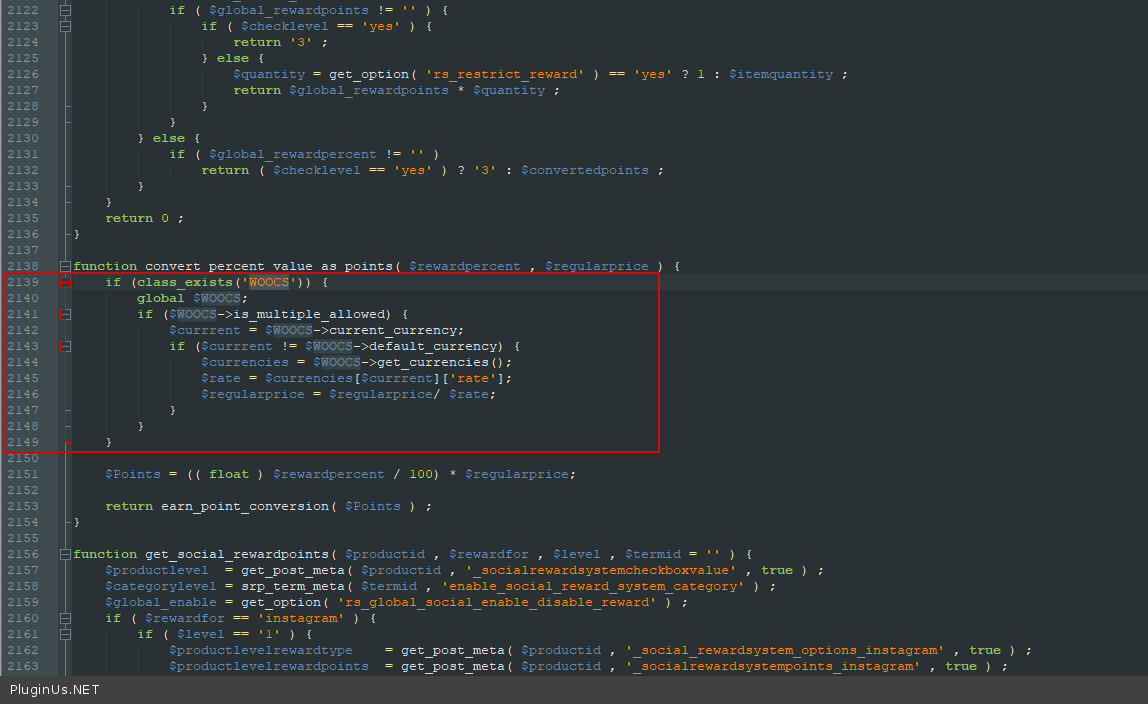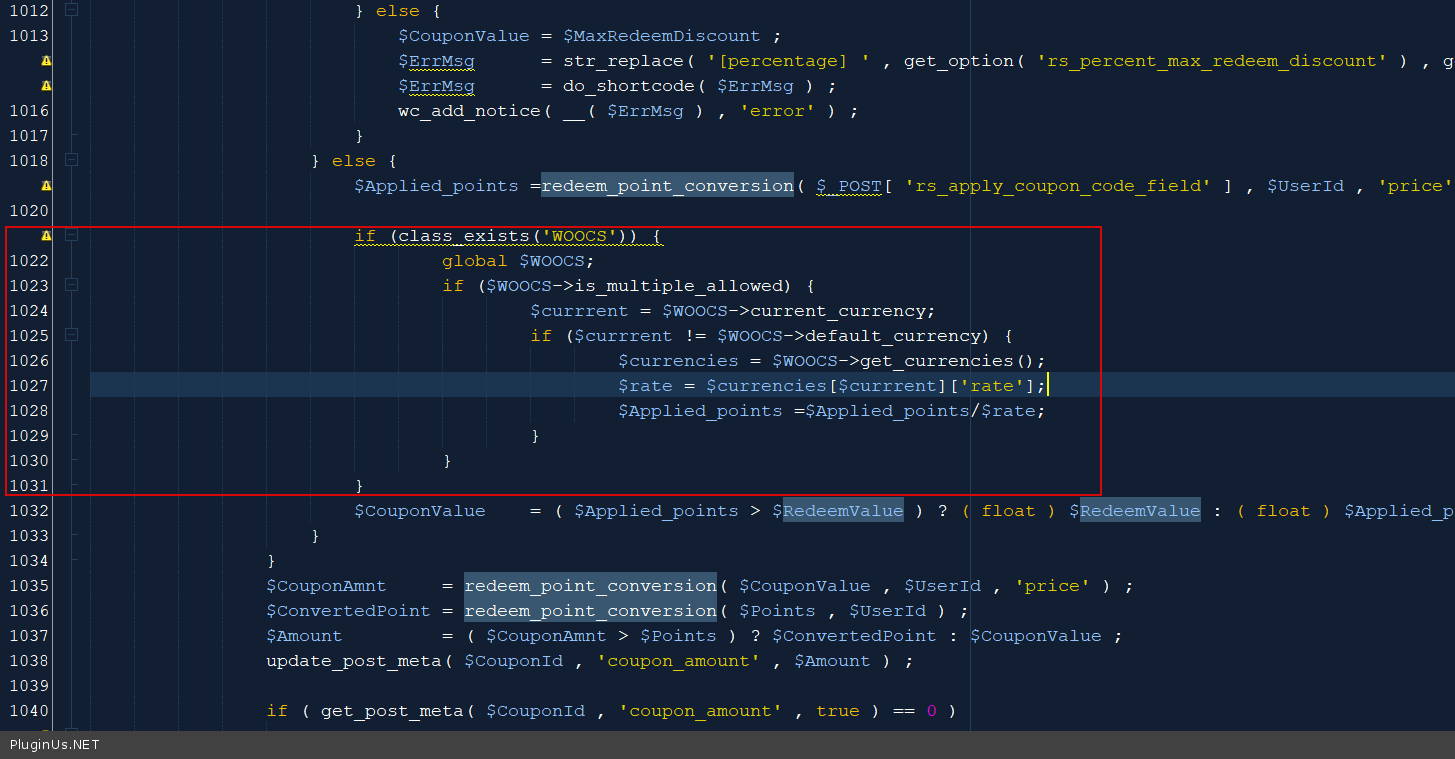# FOX - Currency Switcher Professional for WooCommerce

### SUMO Reward Points – WooCommerce Reward System

• in file wp-content\plugins\rewardsystem\includes\class-fp-common-functions.php add code:
```if (class_exists('WOOCS') AND \$Type != 'price') {
global \$WOOCS;
if (\$WOOCS->is_multiple_allowed) {
\$currrent = \$WOOCS->current_currency;
if (\$currrent != \$WOOCS->default_currency) {
\$currencies = \$WOOCS->get_currencies();
\$rate = \$currencies[\$currrent]['rate'];

\$Value = round(\$Value / \$rate, 2);
}
}
}

\$ConvertedValue = (\$Type == 'price') ? (((float) \$Value / \$PointValue) * \$RedeemPercent) : (((float) \$Value * \$PointValue) / \$RedeemPercent); //Ex:10 * 2 = 20

if (class_exists('WOOCS') AND \$Type == 'price') {
global \$WOOCS;
if (\$WOOCS->is_multiple_allowed) {
\$currrent = \$WOOCS->current_currency;
if (\$currrent != \$WOOCS->default_currency) {
\$currencies = \$WOOCS->get_currencies();
\$rate = \$currencies[\$currrent]['rate'];
\$ConvertedValue = \$ConvertedValue * \$rate;
}
}
}```
```if (class_exists('WOOCS')) {
global \$WOOCS;
if (\$WOOCS->is_multiple_allowed) {
\$currrent = \$WOOCS->current_currency;
if (\$currrent != \$WOOCS->default_currency) {
\$currencies = \$WOOCS->get_currencies();
\$rate = \$currencies[\$currrent]['rate'];
\$regularprice = \$regularprice / \$rate;
}
}
}```• In file wp-content\plugins\rewardsystem\includes\frontend\tab\modules\class-rs-fpredeeming-frontend.php add code:
```if (class_exists('WOOCS')) {
global \$WOOCS;
if (\$WOOCS->is_multiple_allowed) {
\$currrent = \$WOOCS->current_currency;
if (\$currrent != \$WOOCS->default_currency) {
\$currencies = \$WOOCS->get_currencies();
\$rate = \$currencies[\$currrent]['rate'];
\$Applied_points = \$Applied_points / \$rate;
}
}
}```